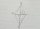# Expression of a variable from formula - examples - page 30

1. Midpoint 5FM=3x-4, MG=5x-26, FG=? Point M is the midpoint of FG. Use the given information to find the missing measure or value.
2. Rectangular plotThe dimensions of a rectangular plot are (x+1)m and (2x-y)m. If the sum of x and y is 3m and the perimeter of the plot is 36m. Find the area of the diagonal of the plot.
3. A kiteABCD is a kite. Angle OBC = 20° and angle OCD = 35°. O is the intersection of diagonals. Find angle ABC, angle ADC and angle BAD.

We apologize, but in this category are not a lot of examples.
Do you have an interesting mathematical example that you can't solve it? Enter it, and we can try to solve it.

To this e-mail address, we will reply solution; solved examples are also published here. Please enter e-mail correctly and check whether you don't have a full mailbox.